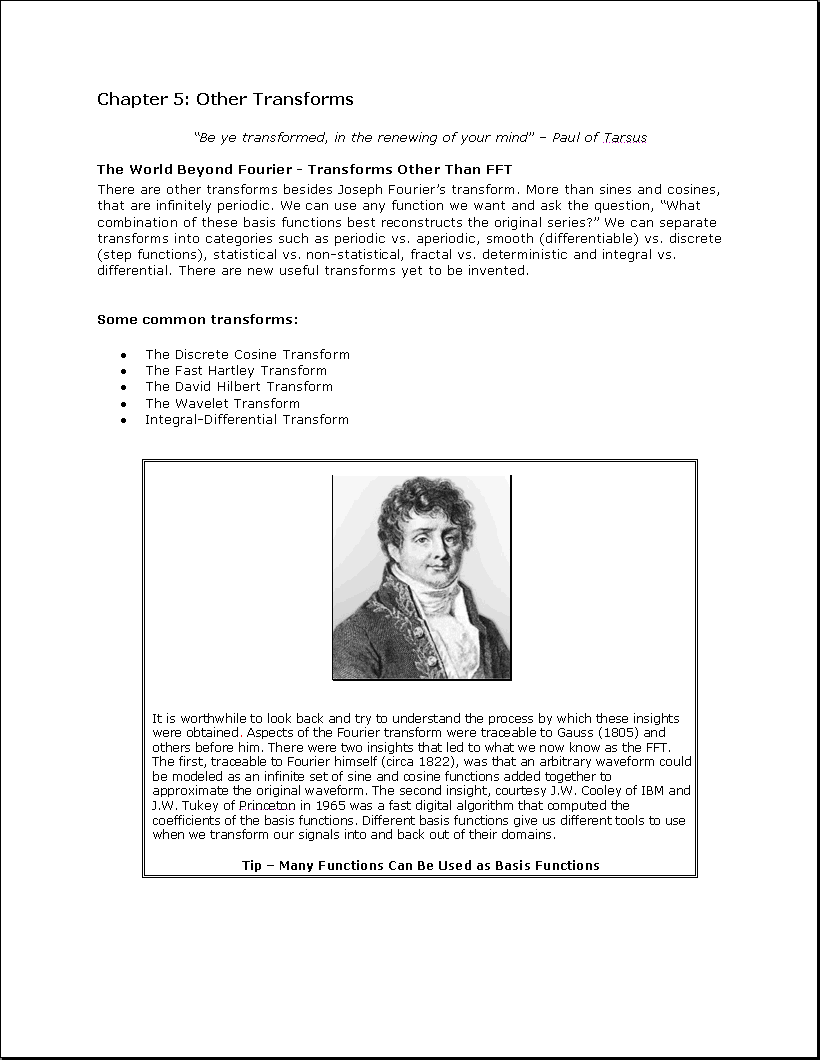Searchable plaintext: Chapter 5: Other Transforms " Be ye transformed, in the renewing of your mind" - Paul of Tarsus The World Beyond Fourier - Transforms Other Than FFT There are other transforms besides Joseph Fourier's transform. More than sines and cosines, that are infinitely periodic. We can use any function we want and ask the question, "What combination of these basis functions best reconstructs the original series?" We can separate transforms into categories such as periodic vs. aperiodic, smooth (differentiable) vs. discrete (step functions), statistical vs. non-statistical, fractal vs. deterministic and integral vs. differential. There are new useful transforms yet to be invented. Some common transforms: * The Discrete Cosine Transform * The Fast Hartley Transform * The David Hilbert Transform * The Wavelet Transform * Integral-Differential Transform It is worthwhile to look back and try to understand the process by which these insights were obtained. Aspects of the Fourier transform were traceable to Gauss (1805) and others before him. There were two insights that led to what we now know as the FFT. The first, traceable to Fourier himself (circa 1822), was that an arbitrary waveform could be modeled as an infinite set of sine and cosine functions added together to approximate the original waveform. The second insight, courtesy J.W. Cooley of IBM and J.W. Tukey of Princeton in 1965 was a fast digital algorithm that computed the coefficients of the basis functions. Different basis functions give us different tools to use when we transform our signals into and back out of their domains. Tip - Many Functions Can Be Used as Basis Functions